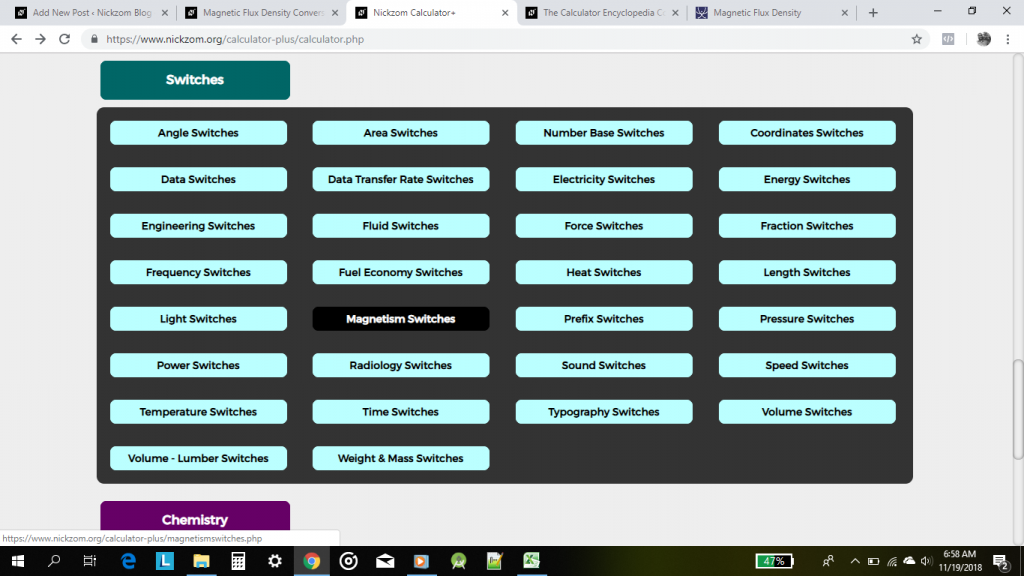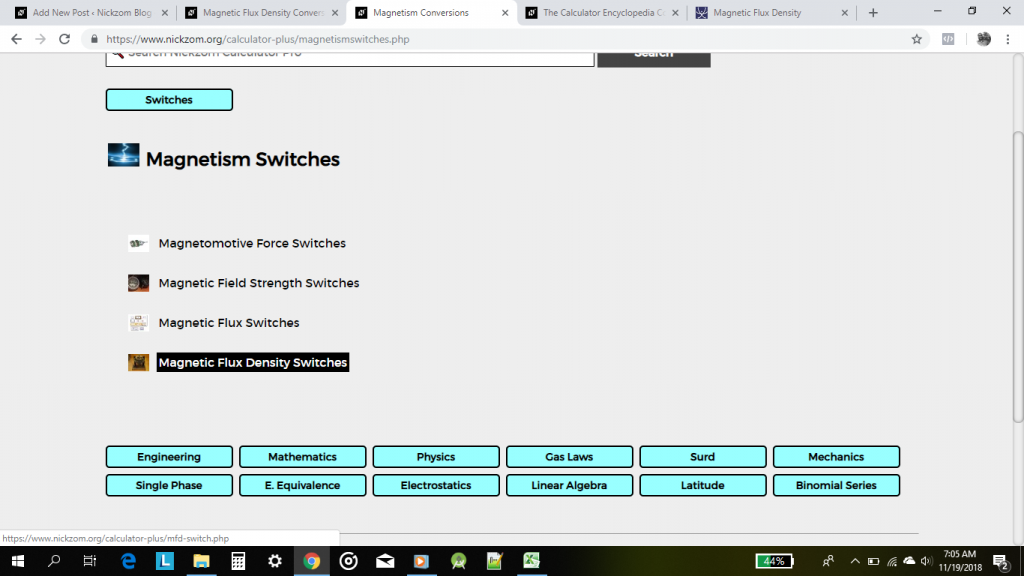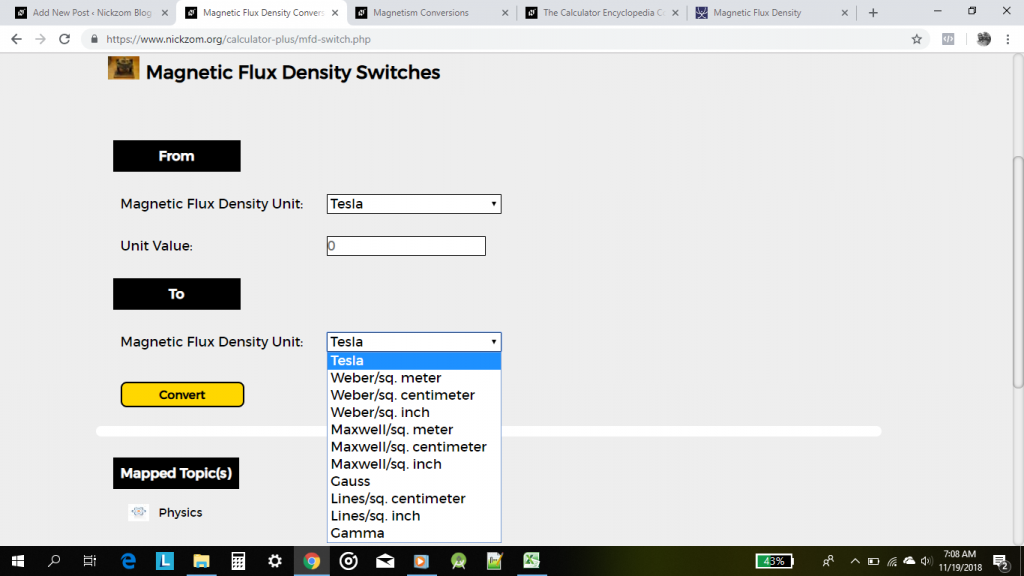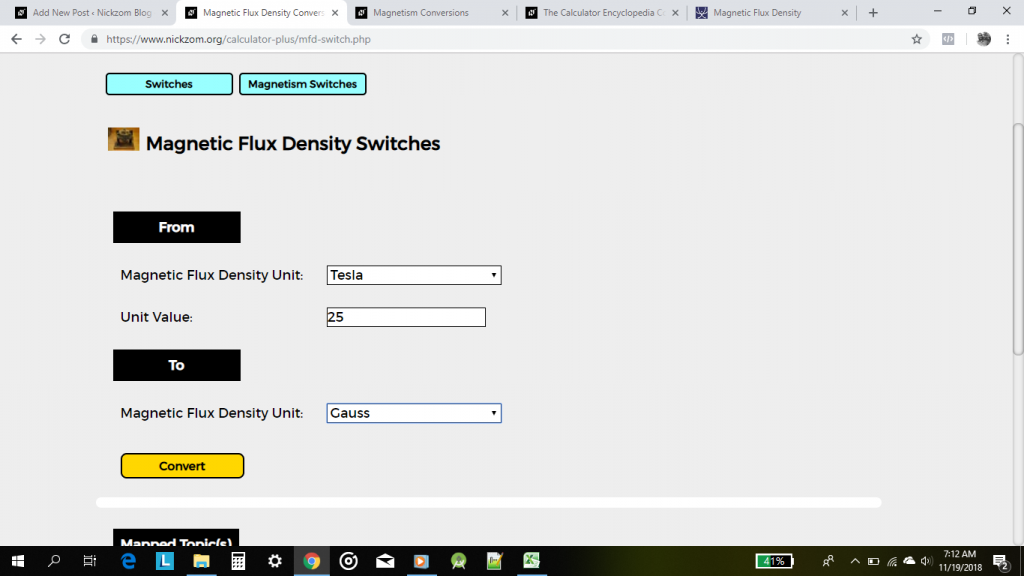# The Calculator Encyclopedia Converts Magnetic Flux Density Units on Magnetism Unit Conversions

According to Mini Physics,

Magnetic flux density (B) is defined as the force acting per unit current per unit length on a wire placed at right angles to the magnetic field.Nickzom Calculator converts 11 units of magnetic flux density such as:

• Tesla
• Weber/sq. meter
• Weber/sq. centimeter
• Weber/sq. inch
• Maxwell/sq. meter
• Maxwell/sq. centimeter
• Maxwell/sq. inch
• Gauss
• Lines/sq. centimeter
• Lines/sq. inch
• Gamma

On Clicking on Magnetism Switches, there are 4 major magnetism unit conversion sections such as:

• Magnetomotive Force Switches
• Magnetic Field Strength Switches
• Magnetic Flux Switches
• Magnetic Flux Density SwitchesFor the purpose of this post, our focus would be on Magnetic Flux Density SwitchesLet’s convert 25 Tesla to Gauss

Enter the unit value and select the appropriate units for the conversionLastly, click on Convert buttonThere goes the answer for the unit conversion between Tesla and Gauss.

One can access Nickzom Calculator+ (Professional Version) – The Calculator Encyclopedia via any of these channels:

## 2 thoughts on “The Calculator Encyclopedia Converts Magnetic Flux Density Units on Magnetism Unit Conversions”

1.camera wifi không dây says:

2.nicolialia pizzeria says: